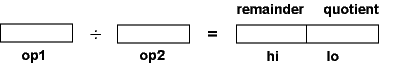```99 / 2   =  49 R 1
99 / 50  =   1 R 49
```

# The ` div ` and the ` divu ` Instructions

With N-digit integer division there are two results, an N-digit quotient and an N-digit remainder. With 32-bit operands there will be (in general) two 32-bit results. MIPS uses the hi and lo registers for the results:Here are the MIPS instructions for integer divide. The "u" means operands and results are in unsigned binary.

```div    s,t        #  lo <— s div t
#  hi <— s mod t
#  operands are two's complement
```
```divu   s,t        #  lo <— s div t
#  hi <— s mod t
#  operands are unsigned
```

### QUESTION 9:

(Review:) What instruction would be used to move the quotient into register `\$8`?